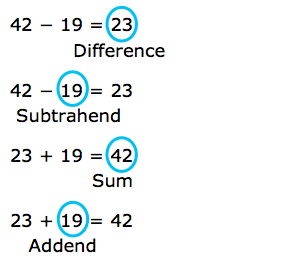Minuend

My Definition

Key Characteristics

The following are true of a minuend:

• A minuend is any number from which another number or quantity is subtracted.

• Minuends may be whole numbers, fractions, decimals, integers, real numbers, and imaginary numbers.

• Minuends may be polynomials.

Example

Use the link below to further study examples of a minuend.

Minuend Activity

Non-example

Difference, subtrahend, sum, and addend.TEKS: This term is not explicitly covered in the Mathematics TEKS.